# Long-Term EU and US GDP Growth Rate by Region, Fourth Quarter 2020

Jun 16, 2021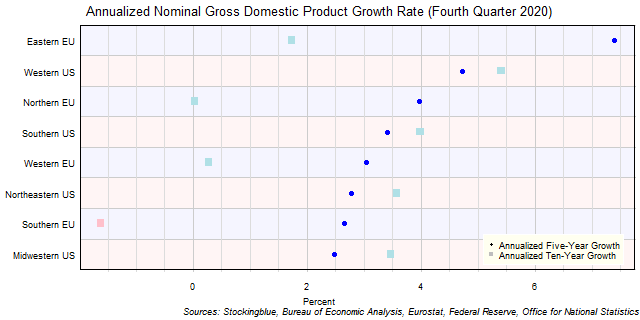The chart above shows the annualized nominal gross domestic product (GDP) growth rate when priced in US dollars in each EU and US region over the past five years as of the fourth quarter of 2020 and the growth over the past ten years.  The Southern EU was the only region that did not experience growth over the past ten years.

# Long-Term EU GDP Growth Rate by Region, Fourth Quarter 2020

Jun 15, 2021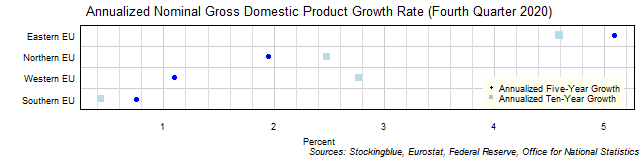The chart above shows the annualized nominal gross domestic product (GDP) growth rate in each EU region over the past five years as of the fourth quarter of 2020 and the growth rate over the past ten years.  All four regions grew over both time periods.

# Long-Term US GDP Growth Rate by Region, Fourth Quarter 2020

Jun 14, 2021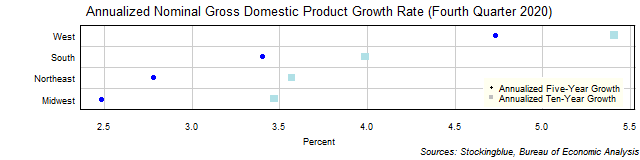The chart above shows the annualized nominal gross domestic product (GDP) growth rate in each US region over the past five years as of the fourth quarter of 2020 and the growth rate over the past ten years.  All four regions grew over both time periods.

# Long-Term EU and US GDP Growth Rate by Region, Third Quarter 2020

Jun 11, 2021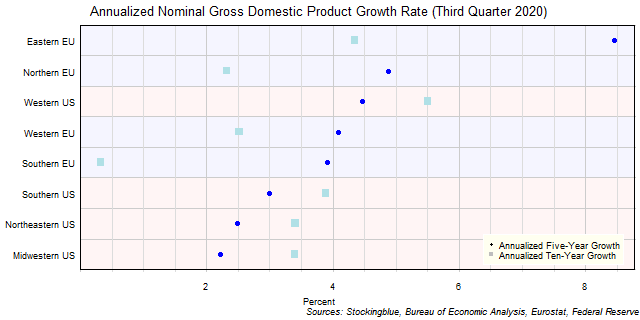The chart above shows the annualized nominal gross domestic product (GDP) growth rate when priced in US dollars in each EU and US region over the past five years as of the third quarter of 2020 and the growth over the past ten years.  All eight regions grew over both time periods.

# Long-Term EU GDP Growth Rate by Region, Third Quarter 2020

Jun 10, 2021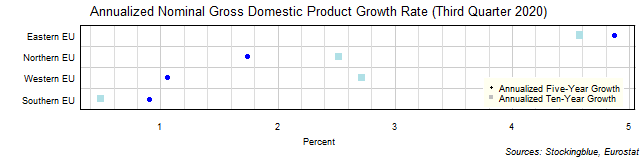The chart above shows the annualized nominal gross domestic product (GDP) growth rate in each EU region over the past five years as of the third quarter of 2020 and the growth rate over the past ten years.  All four regions grew over both time periods.

# Long-Term US GDP Growth Rate by Region, Third Quarter 2020

Jun 9, 2021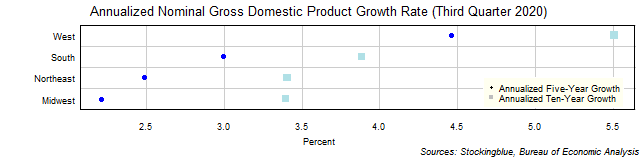The chart above shows the annualized nominal gross domestic product (GDP) growth rate in each US region over the past five years as of the third quarter of 2020 and the growth rate over the past ten years.  All four regions grew over both time periods.

# Long-Term EU and US GDP Growth Rate by State, Fourth Quarter 2020

Jun 4, 2021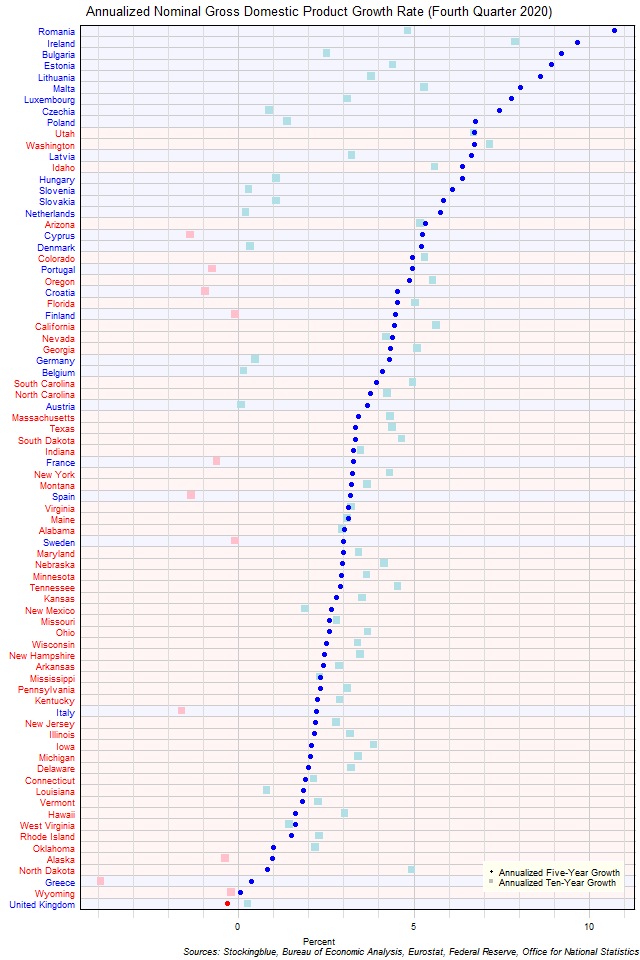The chart above shows the annualized nominal gross domestic product (GDP) growth rate when the GDP is priced in US dollars in each EU and US state over the past five years as of the fourth quarter of 2020 and the growth rate over the past ten years.  Eleven states had negative growth over the past ten years while one had negative growth over the past five.

# Long-Term EU GDP Growth Rate by State, Fourth Quarter 2020

Jun 3, 2021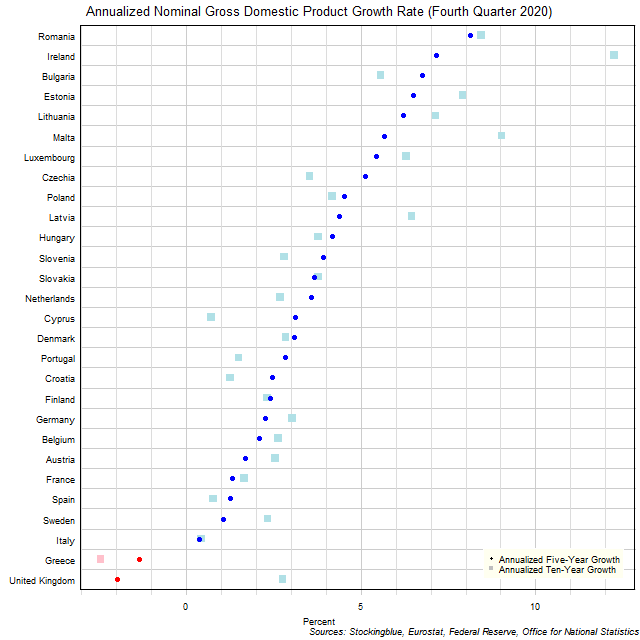The chart above shows the annualized nominal gross domestic product (GDP) growth rate in each EU state over the past five years as of the fourth quarter of 2020 and the growth rate over the past ten years.  Two states experienced negative growth either in the past five years or in the past ten years.

# Long-Term US GDP Growth Rate by State, Fourth Quarter 2020

Jun 2, 2021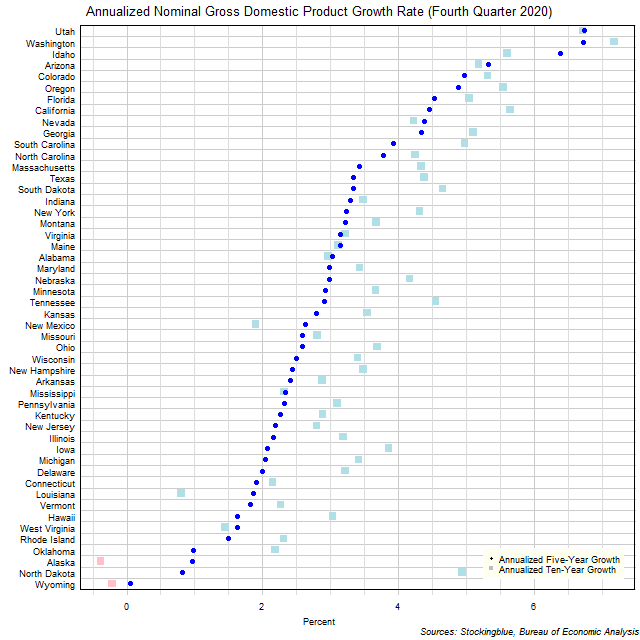The chart above shows the annualized nominal gross domestic product (GDP) growth rate in each US state over the past five years as of the fourth quarter of 2020 and the growth rate over the past ten years.  Two states experienced negative growth either in the past ten years.

# Long-Term Per Capita GDP by EU and US Region, Fourth Quarter 2020

Jun 1, 2021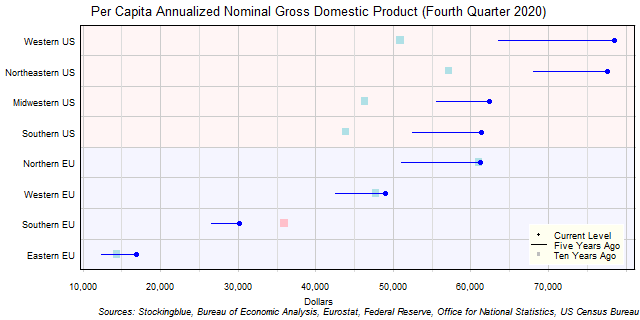The chart above shows the per capita annualized nominal gross domestic product (GDP) in each EU and US region as of the fourth quarter of 2020 in US dollars, the change from five years ago, and the per capita GDP ten years prior.  Only one EU region has seen a drop in per capita GDP over the past five or ten years.

Older